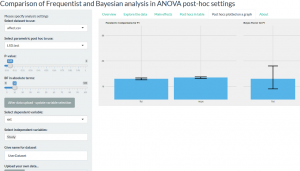### Bayesian Statistics – the Frog Example

An introduction to Bayesian statistics.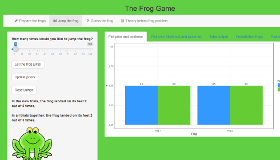### Bayesian t-Test Teaching App

The goal of the Bayesian t-test app is to provide teachers with a handy tool to show students what a Bayes factor, and more generally, what the results from a Bayesian t-test look like when data points are added in real-time.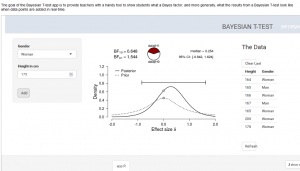### Bayesian Correlation Analysis

The “Bayesian correlation analysis” app shows how the results of Bayesian procedures vary in the light of changes regarding data and prior beliefs.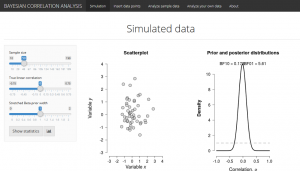### James-Stein-Estimator

The goal of this shiny app is to visualize the effect of shrinkage estimators and compare their performance to other estimators.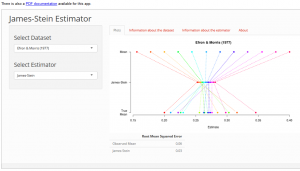### P-Values & Bayes Factors

This app illustrates the relation between p values and Bayes factors for various widely-used statistical tests of hypotheses.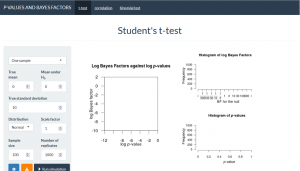### Sequential Testing with p-Values and Bayes Factors

This app illustrates the relation between p-values and Bayes factors for various statistical tests under sequential and block sampling procedures.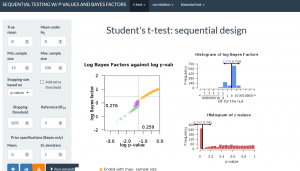### Comparison of Frequentist and Bayesian analysis in ANOVA post-hoc settings